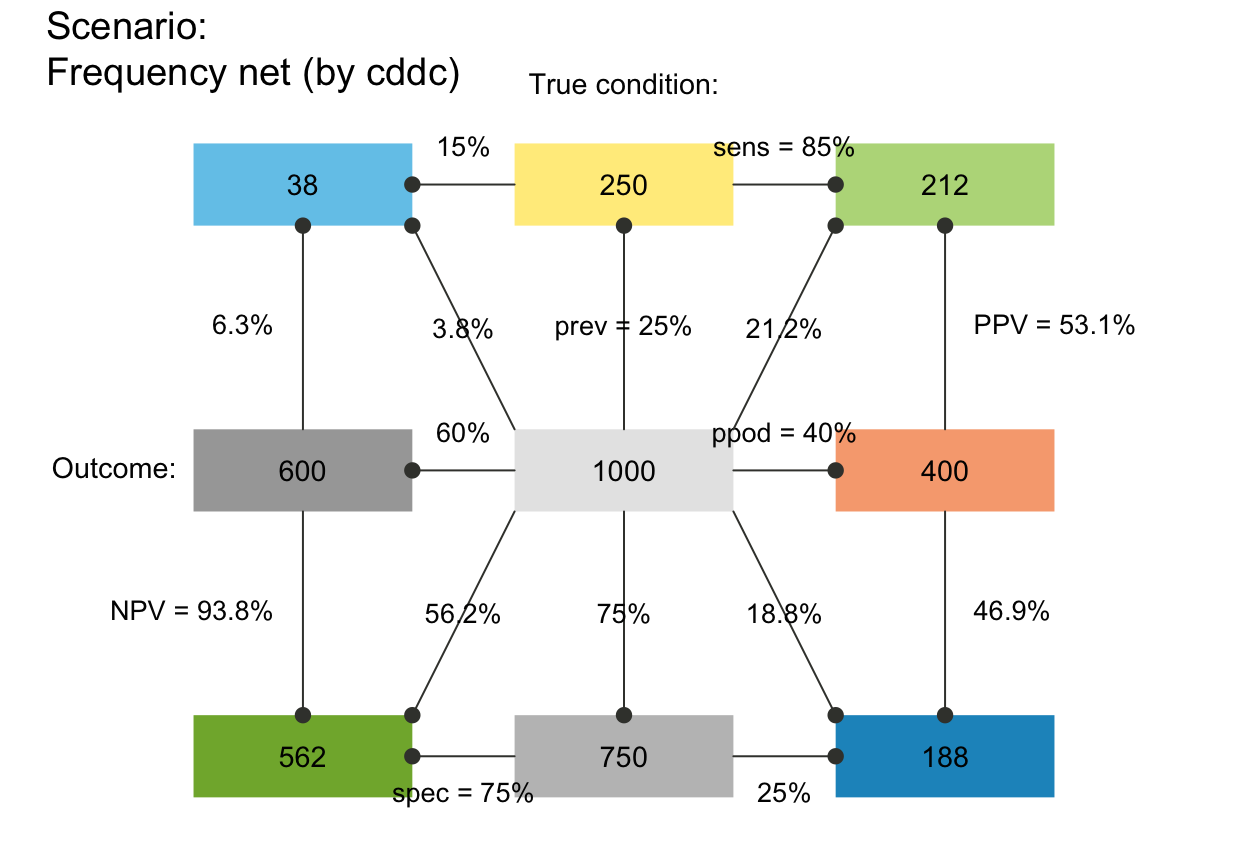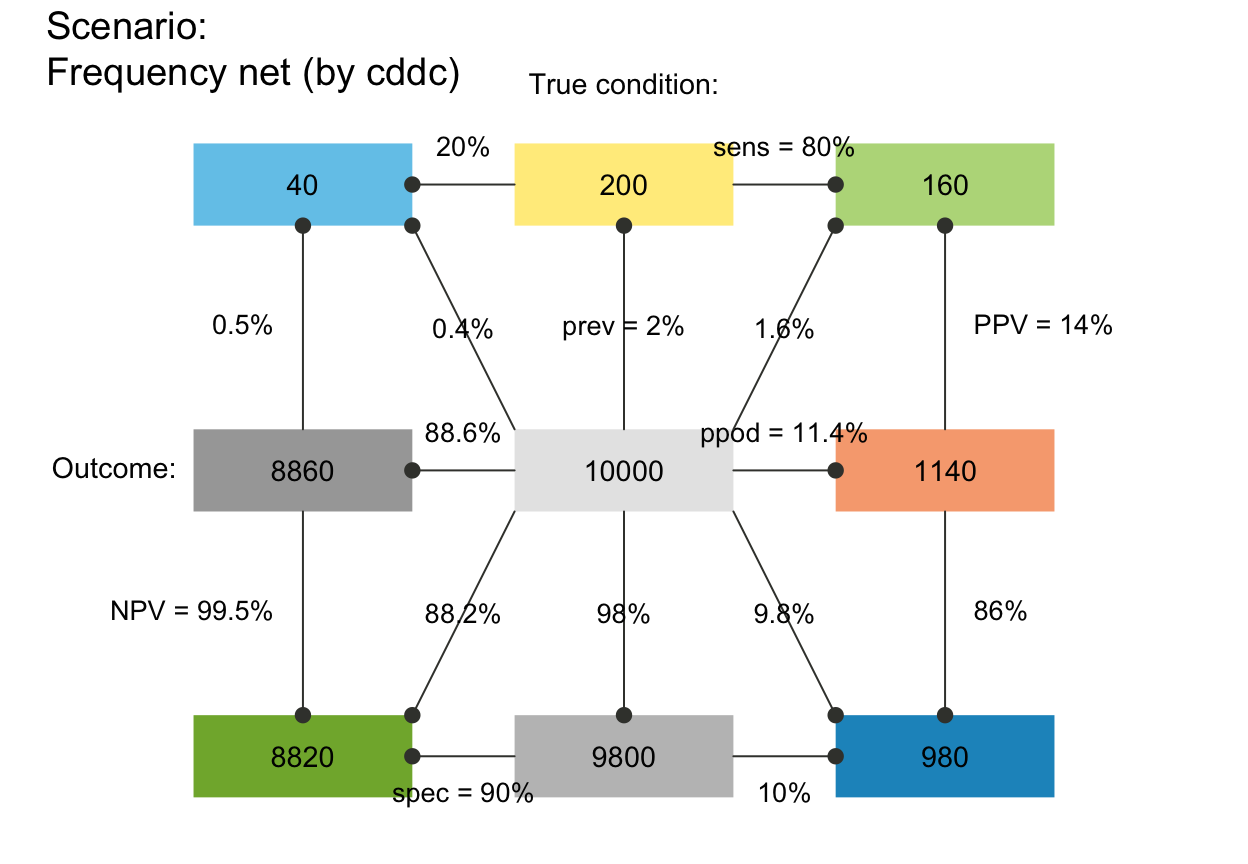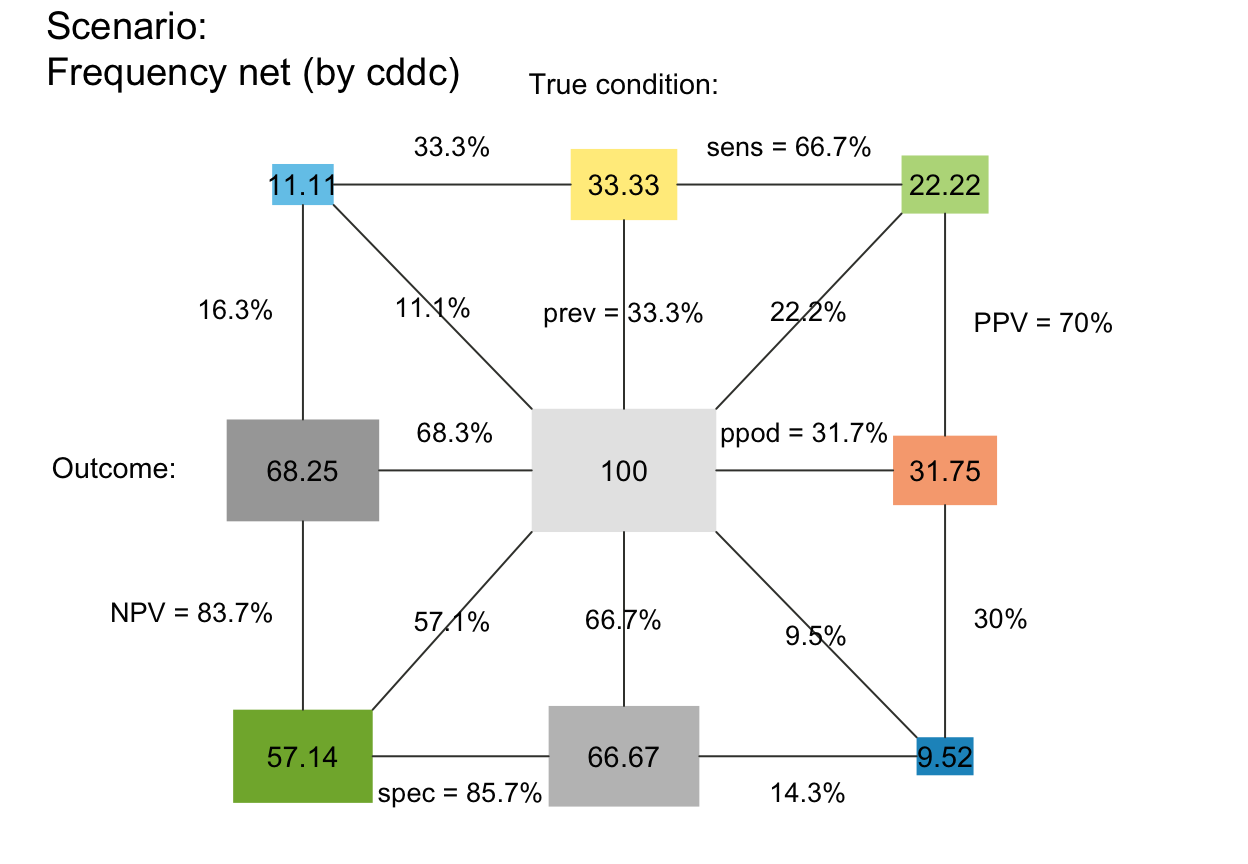plot_fnet drew a network diagram of frequencies (as nodes) and probabilities (as edges).

plot_fnet(prev = num$prev, sens = num$sens, mirt = NA,
spec = num$spec, fart = NA, N = freq$N, round = TRUE,
by = "cddc", area = "no", p_lbl = "num", show_accu = TRUE,
w_acc = 0.5, title_lbl = txt$scen_lbl, popu_lbl = txt$popu_lbl,
cond_true_lbl = txt$cond_true_lbl, cond_false_lbl = txt$cond_false_lbl, dec_pos_lbl = txt$dec_pos_lbl, dec_neg_lbl = txt$dec_neg_lbl, hi_lbl = txt$hi_lbl, mi_lbl = txt$mi_lbl, fa_lbl = txt$fa_lbl, cr_lbl = txt$cr_lbl,
col_txt = grey(0.01, alpha = 0.99), cex_lbl = 0.85,
col_boxes = pal, col_border = grey(0.33, alpha = 0.99), lwd = 1.5,
box_lwd = 1.5, col_shadow = grey(0.11, alpha = 0.99),
cex_shadow = 0)

## Arguments

prev The condition's prevalence prev. The decision's sensitivity sens. The decision's miss rate mirt. The decision's specificity value spec. The decision's false alarm rate fart. The number of individuals in the population. A Boolean option specifying whether computed frequencies are rounded to integers. Default: round = TRUE. A character code specifying the perspective (or categories by which the population is split into subsets) with 3 options: "cddc" ... 1st by condition, 2nd by decision; "dccd" ... 1st by decision, 2nd by condition; "cdac" ... 1st by condition, 2nd by accuracy. A character code specifying the area of the boxes (or their relative sizes) with 3 options: "no" ... all boxes are shown with the same size; "sq" ... boxes are squares with area sizes scaled proportional to frequencies (default); "hr" ... boxes are horizontal rectangles with area sizes scaled proportional to frequencies. A character code specifying the type of probability information (on edges) with 4 options: "nam" ... names of probabilities; "num" ... numeric values of probabilities (rounded to 3 decimals, default); "mix" ... names of essential probabilities, values of complements; "min" ... minimal labels: names of essential probabilities. Option for showing current accuracy metrics accu on the margin of the plot. Weighting parameter w used to compute weighted accuracy w_acc in comp_accu_freq. Various other options allow the customization of text labels and colors: Text label for current plot title. Text label for current population popu. Text label for current cases of cond_true. Text label for current cases of cond_false. Text label for current cases of dec_pos. Text label for current cases of dec_neg. Text label for hits hi. Text label for misses mi. Text label for false alarms fa. Text label for correct rejections cr. Color for text labels (in boxes). Scaling factor for text labels (in boxes and on arrows). Colors of boxes (a single color or a vector with named colors matching the number of current boxes). Default: Current color information contained in pal. Color of borders. Default: col_border = grey(.33, alpha = .99). Width of arrows. Width of boxes. Color of box shadows. Default: col_shadow = grey(.11, alpha = .99). Scaling factor of shadows (values > 0 showing shadows). Default: cex_shadow = 0.

Nothing (NULL).

## Details

plot_fnet is deprecated -- please use plot_prism instead.

plot_prism is the new version of this function.
Other visualization functions: plot.riskyr, plot_area, plot_bar, plot_curve, plot_icons, plot_mosaic, plot_plane, plot_prism, plot_tab, plot_tree
plot_fnet()  # frequency network with default options (by = "cddc")#> Function 'plot_fnet' is deprecated; using 'plot_prism' instead.plot_tree(by = "cdac")  # frequency network by condition and accuracy#> Function 'plot_tree' is deprecated; using 'plot_prism' instead.plot_fnet(by = "dccd")  # frequency network by decision and condition#> Function 'plot_fnet' is deprecated; using 'plot_prism' instead.